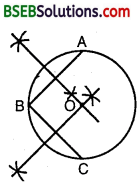# Bihar Board Class 9th Maths Solutions Chapter 10 Circles Ex 10.3

Bihar Board Class 9th Maths Solutions Chapter 10 Circles Ex 10.3 Textbook Questions and Answers.

## BSEB Bihar Board Class 9th Maths Solutions Chapter 10 Circles Ex 10.3Question 1.
Draw different pairs of circles. How many points does each pair have in common? What is the maximum number of common points?
Solution:
On drawing a different pairs of circles, we find that they have two points in common. Now, we shall prove that a pair of circle cannot intersect each other at more than two points. Let the two circles cut each other at three points. But from the three points only one circle passes.

Hence, two circles if intersect each other then they will intersect at two points. These two points are the ends of their common chords. This chord can be a common chord of a number of circles which will pass through the end points of this common chord.Question 2.
Suppose you are given a construction to find its centre.
Solution:
Steps of Construction :
1. Take 3 points A, B and C on the circumference of the circle.
2. Join AB and BC.3. Draw PQ and RS, the perpendicular bisectors of AB and BC, which intersect each other at O. Then, O is the centre of the circle.Question 3.
If two circles intersect at two points, prove that their centres lie on the perpendicular bisector of the common chord.
Solution:
Given : Two circles, with centres O and O’ intersect at two points A and B so that AB is the common chord of the two circles and 00′ is the line segment joining the centres of the two circles. Let OO’ intersect AB at M.
To prove : OO’ is the perpendicular bisector of AB.
Construction : Draw line segments OA, OB, O’A and O’B.Proof:
In As OAO’ and OBO
OA = OB [Radii of the same circle]
O’A = O’B [Radii of the same circle]
and; OO’ = OO’
By SSS criterion of congruence, we have
∆ OAO’ ≅ ∆ OBO’
⇒ ∠AO O’ = ∠BOO’
⇒ ∠AOM = ∠BOM …(1) [∵ ∠AOO’ = ∠AOM and ∠BOM = ∠BOO’]
In ∆s AOM and BOM, we have
OA = OB [Radii of the same circle]
∠AOM = ∠BOM [From (1)]
and, OM = OM [Common]
∴ By SAS criterion of congruence, we have
∆ AOM ≅ ∆ BOM
⇒ AM = BM and ∠AMO = ∠BMO
But ∠AMO + ∠BMO = 180°
∴ ∠AM0 = 180° ⇒ ∠AMO = 90°
Thus, AM = BM and ∠AMO = ∠BMO = 90°
Hence, OO’ is the perpendicular bisector of AB.